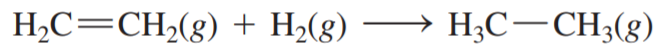×
Get Full Access to Chemistry: A Molecular Approach - 3 Edition - Chapter 9 - Problem 81e
Get Full Access to Chemistry: A Molecular Approach - 3 Edition - Chapter 9 - Problem 81e

×

# Hydrogenation reactions are used to add hydrogen acrossISBN: 9780321809247 1

## Solution for problem 81E Chapter 9

Chemistry: A Molecular Approach | 3rd Edition

• Textbook Solutions
• 2901 Step-by-step solutions solved by professors and subject experts
• Get 24/7 help from StudySoup virtual teaching assistantsChemistry: A Molecular Approach | 3rd Edition

4 5 1 411 Reviews
31
4
Problem 81E

Hydrogenation reactions are used to add hydrogen across double bonds in hydrocarbons and other organic compounds. Use average bond energies to calculaterxn for the hydrogenation reaction.Step-by-Step Solution:
Step 1 of 3

Solution:Step-1 + -The electronic configuration of CN , CN and CN ions, can be predicted from its MO electronicconfigurationTotal number of electrons in CN = 6+7=13Hence the electronic configuration = 1s *1s 2s *2s 2p 2 2 2 x2p y 2p z - Total number of electrons in CN ion= 6+7+1=14Hence the electronic configuration = 1s *1s 2s *2s 2p 2 2 2 22p 2 2p 2 x y zTotal number of electrons in CN ion= 6+7-1=12+ 2 2 2 2 2 2Hence the electronic configuration = 1s *1s 2s *2s 2p x 2p yStep-2(a)Bond order of CN = number of electron in bonding atomic orbital number of electron in antibonding orbital 2 = 94 =5/2=2.5 2Bond order of CN ion = - number of electron in bonding atomic orbital number of electron in antibonding orbital 2 = 104 =6/2=3 2 number of electron in bonding atomic orbital number of electron in antibonding orbitalBond order of CN ion = + 84 2 = 2 =4/2=2As it is known that the bond order is directly proportional to the bond strength i.e higher thebond order stronger will be the bond strength.Thus the order of bond strength will be, CN > CN >CN ion - + Step-3(b) Among all these molecules, CN has only 1 unpaired electron and hence it is paramagnetic.

Step 2 of 3

Step 3 of 3

##### ISBN: 9780321809247

The full step-by-step solution to problem: 81E from chapter: 9 was answered by , our top Chemistry solution expert on 02/22/17, 04:35PM. The answer to “?Hydrogenation reactions are used to add hydrogen across double bonds in hydrocarbons and other organic compounds. Use average bond energies to calculate rxn for the hydrogenation reaction.” is broken down into a number of easy to follow steps, and 27 words. Chemistry: A Molecular Approach was written by and is associated to the ISBN: 9780321809247. This textbook survival guide was created for the textbook: Chemistry: A Molecular Approach, edition: 3. Since the solution to 81E from 9 chapter was answered, more than 714 students have viewed the full step-by-step answer. This full solution covers the following key subjects: hydrogenation, hydrocarbons, average, Bond, bonds. This expansive textbook survival guide covers 82 chapters, and 9454 solutions.

## Discover and learn what students are asking

Calculus: Early Transcendental Functions : Preparation for Calculus
?In Exercises 1–4, find any intercepts. $$y=(x-3) \sqrt{x+4}$$

Statistics: Informed Decisions Using Data : Testing the Significance of the Least-Squares Regression Model
?In Problems 5–10, use the results of Problems 7–12, respectively, from Section 4.2 to answer the following questions: (a) What are the estima

Statistics: Informed Decisions Using Data : Testing the Significance of the Least-Squares Regression Model
?Why don’t we conduct inference on the linear correlation coefficient?

Unlock Textbook Solution